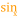# Sine

 Block Group: Trigonometric Functions Icon:The Sine block returns the sine of the given angle.

For information on using dataflow blocks, see Dataflow.

## Input/Output Property

The following property of the Sine block can take input and give output.

• input (number)

input specifies the angle to get the sine for, in degrees.

## Output Property

The following property of the Sine block can give output but cannot take input.

• output (number)

output returns the sine of the input.

## Example

The following image shows an example of the Sine block. In this example, the angle is 90 degrees, and the sine is one.